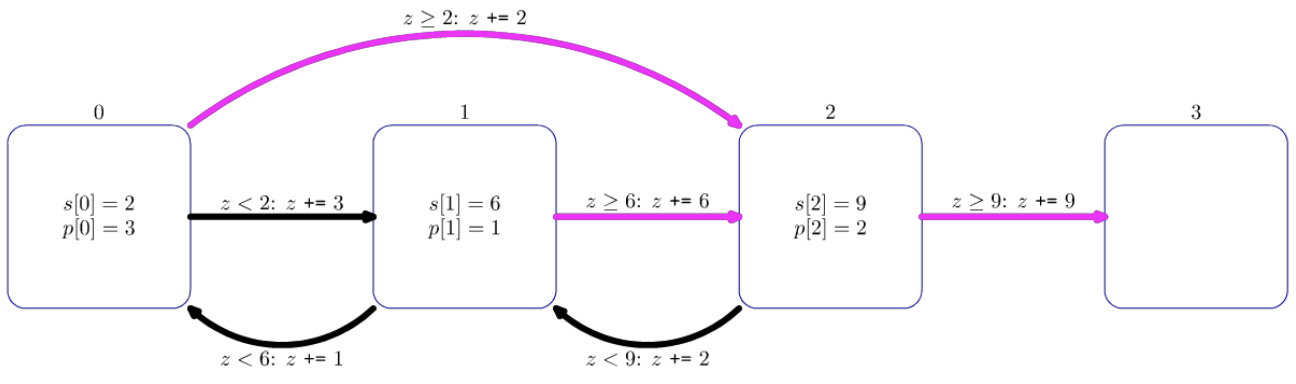시간 제한메모리 제한제출정답맞힌 사람정답 비율
4 초 2048 MB130191815.385%

## 문제

Robert is designing a new computer game. The game involves one hero, $n$ opponents and $n + 1$ dungeons. The opponents are numbered from $0$ to $n - 1$ and the dungeons are numbered from $0$ to $n$. Opponent $i$ ($0 \le i \le n - 1$) is located in dungeon $i$ and has strength $s[i]$. There is no opponent in dungeon $n$.

The hero starts off entering dungeon $x$, with strength $z$. Every time the hero enters any dungeon $i$ ($0 \le i \le n - 1$), they confront opponent $i$, and one of the following occurs:

• If the hero's strength is greater than or equal to the opponent's strength $s[i]$, the hero wins. This causes the hero's strength to increase by $s[i]$ ($s[i] \ge 1$). In this case the hero enters dungeon $w[i]$ next ($w[i] > i$).
• Otherwise, the hero loses. This causes the hero's strength to increase by $p[i]$ ($p[i] \ge 1$). In this case the hero enters dungeon $l[i]$ next.

Note $p[i]$ may be less than, equal to, or greater than $s[i]$. Also, $l[i]$ may be less than, equal to, or greater than $i$. Regardless of the outcome of the confrontation, the opponent remains in dungeon $i$ and maintains strength $s[i]$.

The game ends when the hero enters dungeon $n$. One can show that the game ends after a finite number of confrontations, regardless of the hero's starting dungeon and strength.

Robert asked you to test his game by running $q$ simulations. For each simulation, Robert defines a starting dungeon $x$ and starting strength $z$. Your task is to find out, for each simulation, the hero's strength when the game ends.

## 구현

You should implement the following procedures:

void init(int n, int[] s, int[] p, int[] w, int[] l)
• $n$: number of opponents.
• $s$, $p$, $w$, $l$: arrays of length $n$. For $0 \le i \le n - 1$:
• $s[i]$ is the strength of the opponent $i$. It is also the strength gained by the hero after winning against opponent $i$.
• $p[i]$ is the strength gained by the hero after losing against opponent $i$.
• $w[i]$ is the dungeon the hero enters after winning against opponent $i$.
• $l[i]$ is the dungeon the hero enters after losing against opponent $i$.
• This procedure is called exactly once, before any calls to simulate (see below).
int64 simulate(int x, int z)
• $x$: the dungeon the hero enters first.
• $z$: the hero's starting strength.
• This procedure should return the hero's strength when the game ends, assuming the hero starts the game by entering dungeon $x$, having strength $z$.
• The procedure is called exactly $q$ times.

## 예제

Consider the following call:

init(3, [2, 6, 9], [3, 1, 2], [2, 2, 3], [1, 0, 1])The diagram above illustrates this call. Each square shows a dungeon. For dungeons $0$, $1$ and $2$, the values $s[i]$ and $p[i]$ are indicated inside the squares. Magenta arrows indicate where the hero moves after winning a confrontation, while black arrows indicate where the hero moves after losing.

Let's say the grader calls simulate(0, 1).

The game proceeds as follows:

Dungeon Hero's strength before confrontation Result
$0$ $1$ Lose
$1$ $4$ Lose
$0$ $5$ Win
$2$ $7$ Lose
$1$ $9$ Win
$2$ $15$ Win
$3$ $24$ Game ends

As such, the procedure should return $24$.

Let's say the grader calls simulate(2, 3).

The game proceeds as follows:

Dungeon Hero's strength before confrontation Result
$2$ $3$ Lose
$1$ $5$ Lose
$0$ $6$ Win
$2$ $8$ Lose
$1$ $10$ Win
$2$ $16$ Win
$3$ $25$ Game ends

As such, the procedure should return $25$.

## 제한

• $1 \le n \le 400\,000$
• $1 \le q \le 50\,000$
• $1 \le s[i], p[i] \le 10^7$ (for all $0 \le i \le n - 1$)
• $0 \le l[i], w[i] \le n$ (for all $0 \le i \le n - 1$)
• $w[i] > i$ (for all $0 \le i \le n - 1$)
• $0 \le x \le n - 1$
• $1 \le z \le 10^7$

## 서브태스크

번호배점제한
111

$n \le 50\,000$, $q \le 100$, $s[i], p[i] ≤ 10\,000$ (for all $0 \le i \le n - 1$)

226

$s[i] = p[i]$ (for all $0 \le i \le n - 1$)

313

$n \le 50\,000$, all opponents have the same strength, in other words, $s[i] = s[j]$ for all $0 \le i, j \le n - 1$.

412

$n \le 50\,000$, there are at most $5$ distinct values among all values of $s[i]$.

527

$n \le 50\,000$

611

## 샘플 그레이더

The sample grader reads the input in the following format:

• line $1$: $n$ $q$
• line $2$: $s$ $s$ $\cdots$ $s[n - 1]$
• line $3$: $p$ $p$ $\cdots$ $p[n - 1]$
• line $4$: $w$ $w$ $\cdots$ $w[n - 1]$
• line $5$: $l$ $l$ $\cdots$ $l[n - 1]$
• line $6 + i$ ($0 \le i \le q - 1$): $x$ $z$ for the $i$-th call to simulate.

The sample grader prints your answers in the following format:

• line $1 + i$ ($0 \le i \le q - 1$): the return value of the $i$-th call to simulate.

## 제출할 수 있는 언어

C++17, C++14, C++20, C++14 (Clang), C++17 (Clang), C++20 (Clang)

## 채점 및 기타 정보

• 예제는 채점하지 않는다.